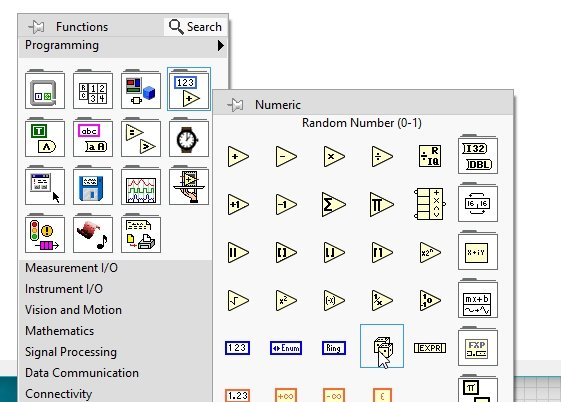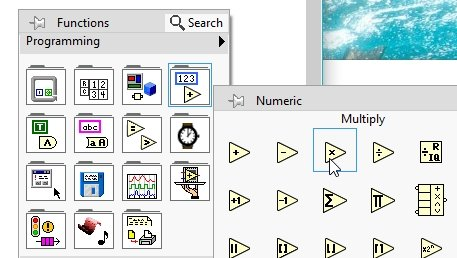# Guess the number game in labview: tutorial 30

In this tutorial we learn something fun. I will guide you to design a game named as “Guess the number”. The user will enter an input number within the range of 0 to 10 as a guess; if the number guessed by the user is correct the LED will blink otherwise it will remain turned off. At the end of the tutorial you are provided with an exercise to do it by yourself, and in the next tutorials I will assume that you have done those exercises and I will not explain the concept regarding them.

### Guess the number game in labview

• Now lets’ design a VI performing the operation described above. First of all, create a VI as we have done in tutorial 1 and save it for future use as we have been doing in the entire previous tutorial.
• The VI we are going to design will generate random numbers within the range of 0 to 10 and it will compare the input value of the user with the randomly generated number, if they are same the indicator LED will turn ON otherwise it will remain OFF.
• From the function palette on the block diagram, select numeric and then select Random Number as shown in the figure below,Figure 1: Random generator

• The problem here is, the built in random number generator generates random number within the range of 0-1 and it generates floating point random numbers, where as we want to compare it with integers. Hence, first we have to manipulate the range of random number generator somehow to 0-10.
• We can do this simply by multiply the output of the random number generator by 10. From the function palette on the block diagram, select numeric and then select multiply as shown in the figure below,Figure 2: Multiply block

Figure 3: Constant placement

Figure 4: Floating random number

Figure 5: Using to integer block

Figure 6: Indicator creation

Figure 7: Creating control

Figure 8: Comparison block placement

Figure 9: Comparison block

Figure 10: LED indicator for correction

Figure 11: Block diagram

Figure 12: Correct guess

Figure 13: Guess is incorect

Figure 15: Correct guess

Exercise:

• Create a guess number game that will allow you to guess numbers between the range 2 -8 instead of 0 to 10.

<< Previous tutorial                                            Next tutorial>>